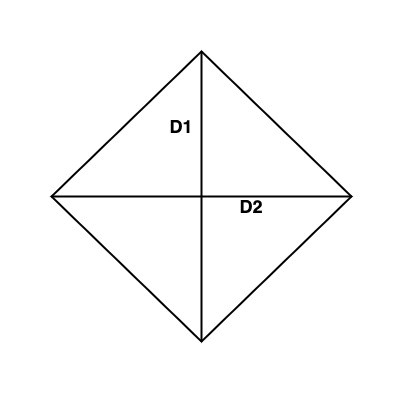# Swift Program to calculate the area of the rhombus

This tutorial will discuss how to write a Swift program to calculate the area of the rhombus.A rhombus is a closed two-dimensional figure or we can say that a rhombus is a quadrilateral whose all the sides are equal and the diagonals intersect each other at a right angle(that is 90 degrees).

The area of the rhombus is known as the total space enclosed inside the boundaries of the rhombus. We can calculate the area of the rhombus using any of the following methods −

• Area of rhombus using diagonal

• Area of rhombus using base and height

• Area of rhombus using trigonometry

## Area of rhombus using diagonal

We can calculate the area of the rhombus using diagonals. The area is equal to half of the product of the length of both diagonals.

### Formula

Following is the formula of the area of the rhombus −

Area = 1/2 x (diagonal1) x (diagonal2)


### Algorithm

Following is the algorithm −

• Step 1 − Declare two variables to store the value of height and base of the rhombus. Here the height and base of the rhombus can be user-defined or pre-defined.

• Step 2 − Declare another variable named as “areaRhombus” to store the area of the rhombus.

var areaRhombus = (rDiagonal1 * rDiagonal2) / 2

• Step 3 − Print the output.

### Example

The following program shows how to calculate the area of the rhombus.

import Foundation
import Glibc

var rDiagonal1 = 6
var rDiagonal2 = 8

// Finding area of rhombus
var areaRhombus = (rDiagonal1 * rDiagonal2) / 2

print("Diagonal 1 - ", rDiagonal1)
print("Diagonal 2 - ", rDiagonal2)
print("Hence the area of Rhombus is", areaRhombus)


### Output

Diagonal 1 -  6
Diagonal 2 -  8
Hence the area of Rhombus is 24


Here, in the above code, we find the area of the rhombus by finding the half of the product of its diagonals using the following code −

var areaRhombus = (rDiagonal1 * rDiagonal2) / 2


Here, rDiagonal1 = 6 and rDiagonal2 = 8, so the area of the rhombus 24((6 * 8)/2 = 24)

## Area of rhombus using base and height

We can also find the area of the rhombus by multiplying the base and height of the rhombus.

### Formula:

Following is the formula of the area of the rhombus −

Area = (base) x (height)


### Algorithm

Following is the algorithm −

• Step 1 − Declare two variables to store the value of height and base of the rhombus. Here the height and base of the rhombus can be user-defined or pre-defined.

• Step 2 − Declare another variable named as “areaRhombus” to store the area of the rhombus.

var areaRhombus = rSideLength * rHeight

• Step 3 − Print the output.

### Example

The following program shows how to calculate the area of the rhombus using base and height.

import Foundation
import Glibc

var rSideLength = 10
var rHeight = 7

// Finding the area of rhombus
var areaRhombus = rSideLength * rHeight

print("Side length - ", rSideLength)
print("Height - ", rHeight)
print("Hence the area of Rhombus is", areaRhombus)


### Output

Side length - 10
Height - 7
Hence the area of Rhombus is 70


Here, in the above code, we find the area of the rhombus by multiplying the base and height of the rhombus using the following code −

var areaRhombus = rSideLength * rHeight


Here, rSideLength = 10 and Height = 7 so the area of the rhombus is 70(10 * 7 = 70).

## Area of rhombus using trigonometry

We can calculate the area of rhombus when the sides and the angle is known with the help of trigonometry. Here we find the area by multiplying the square of side with the sin value of the given angle.

### Formula

Following is the formula of the area of the rhombus −

Area = (base)2 x sin(theta)


### Algorithm

Following is the algorithm −

• Step 1 − Declare a variable to store the side of the rhombus. Here the value can be user-defined or pre-defined.

• Step 2 − Find the radian value of the angle and store the result in rTheta variable.

var rTheta = 90 * (Double.pi / 180)

• Step 3 − Find the area of rhombus and store the result into “areaRhombus” variable.

var areaRhombus = pow(rSideLength, 2) * sin(rTheta)

• Step 4 − Print the output.

### Example

The following program shows how to calculate the area of the rhombus using trigonometry.

import Foundation
import Glibc

var rSideLength = 10.0

// Finding the radian value of the angle
var rTheta = 90 * ( Double.pi / 180 )

// Finding the area of rhombus
var areaRhombus = pow(rSideLength, 2) * sin(rTheta)

print("Side length - ", rSideLength)
print("Hence the area of Rhombus is", areaRhombus)


### Output

Side length - 10.0 Angle(in radian)- 1.5707963267948966
Hence the area of Rhombus is 100.0


Here in the above code, first we find the radian value of the given angle and then we calculate the area of the rhombus using the following code −

var areaRhombus = pow(rSideLength, 2) * sin(rTheta)


Here we use pow() function to calculate the power of side and sin() function is used to calculate the sin value of the given angle. The given side = 10 and angle = 1 so the area of the rhombus is 100.

Updated on: 25-Aug-2022

154 Views